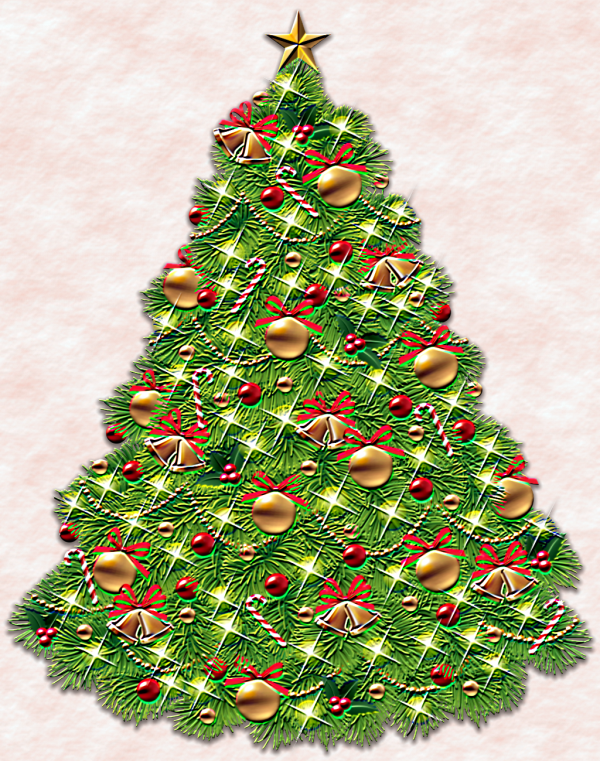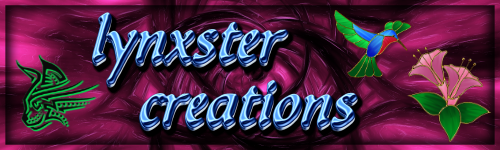# Emboss/Relief+

## Recommended Posts

This is essentially a upgraded version of the existing Paint.NET Emboss/Relief.  Emboss/Relief+ has options such as changing radius, changing internal convolution kernel, and finally, having the option for each channel has their own convolution result rather than utilizing the average of convolution.

The original code was based on this  - https://www.mathworks.com/matlabcentral/fileexchange/9645-steerable-gaussian-filters

Output of Result:Source code

CodeLab:

Spoiler
```// Name: Emboss/Relief+
// Author: Reptillian
// Title: Emboss/Relief+
// Version: 1
// Desc: Extended version of Emboss/Relief
// Keywords: Emboss, Relief
// URL: https://forums.getpaint.net/profile/85868-reptillian/
// Help:

#region UICode
AngleControl var_angle = 90; // [-180,180] Angle
DoubleSliderControl sigma = 0.5; // [0.25,4] Sigma
DoubleSliderControl scale_value = 2; // [0.5,10] Value Scale
ListBoxControl output = 0; // Output|Relief|Emboss
CheckboxControl rgb_mode = true; // RGB Mode
#endregion

double[] convolve_a;
double[] convolve_b;

int cut(int v,int image_max_index){
return Math.Max(0,Math.Min(v,image_max_index));
}

int d2i(double v){
return (int)(Math.Round(v));
}

int average(int r, int g, int b){
return (r+g+b)/3;
}

double tau_sqrt = Math.Sqrt ( 2 * Math.PI);

// This single-threaded function is called after the UI changes and before the Render function is called
// The purpose is to prepare anything you'll need in the Render function
void PreRender(Surface dst, Surface src)
{

double sigma_exp = 2 * sigma * sigma;
double sqrsig = sigma * tau_sqrt;
double M = 3 * sigma;
double ni,rescale_factor;

rescale_factor = 0;

for (int p = 0 ; p < radius ; p++){
ni = ((double)(p)-center_index)/center_index*M;
convolve_a[p] = Math.Exp(-(ni * ni)/sigma_exp)/sqrsig;
convolve_b[p] = (ni / (sigma * sigma)) * convolve_a[p];
rescale_factor +=convolve_a[p];
}

rescale_factor /= scale_value ;

for (int p = 0 ; p < radius ; p++){
convolve_a[p]/=rescale_factor;
convolve_b[p]/=rescale_factor;
}
}

// Here is the main multi-threaded render function
// The dst canvas is broken up into rectangles and
// your job is to write to each pixel of that rectangle
void Render(Surface dst, Surface src, Rectangle rect)
{
double theta = var_angle / 180 * Math.PI;
double cos_theta = Math.Cos(theta);
double sin_theta = Math.Sin(theta);
int max_x = src.Width - 1;
int max_y = src.Height - 1;
double inner_convolve_a_r,inner_convolve_a_g,inner_convolve_a_b,inner_convolve_b_r,inner_convolve_b_g,inner_convolve_b_b;
double outer_convolve_a_r,outer_convolve_a_g,outer_convolve_a_b,outer_convolve_b_r,outer_convolve_b_g,outer_convolve_b_b;
double result_r,result_g,result_b;
int new_r,new_g,new_b,avg;

int start_x,start_y;

ColorBgra Reference_Color_A,Reference_Color_B;

// Step through each row of the current rectangle
for (int y = rect.Top; y < rect.Bottom; y++)
{
if (IsCancelRequested) return;
// Step through each pixel on the current row of the rectangle
for (int x = rect.Left; x < rect.Right; x++)
{
start_x=x-center;
start_y=y-center;

inner_convolve_a_r = inner_convolve_a_g = inner_convolve_a_b = inner_convolve_b_r = inner_convolve_b_g = inner_convolve_b_b = 0;

outer_convolve_a_r = outer_convolve_a_g = outer_convolve_a_b = outer_convolve_b_r = outer_convolve_b_g = outer_convolve_b_b = 0;

for (int p = 0 ; p < radius ; p++)
{
for (int q = 0 ; q < radius ; q++)
{
Reference_Color_A = src[cut(start_x+p,max_x),cut(start_y+q,max_y)];
inner_convolve_a_r += (double)(Reference_Color_A.R) * convolve_a[q];
inner_convolve_a_g += (double)(Reference_Color_A.G) * convolve_a[q];
inner_convolve_a_b += (double)(Reference_Color_A.B) * convolve_a[q];
Reference_Color_B = src[cut(start_x+q,max_x),cut(start_y+p,max_y)];
inner_convolve_b_r += (double)(Reference_Color_B.R) * convolve_a[q];
inner_convolve_b_g += (double)(Reference_Color_B.G) * convolve_a[q];
inner_convolve_b_b += (double)(Reference_Color_B.B) * convolve_a[q];

}

outer_convolve_a_r += inner_convolve_a_r * convolve_b[p];
outer_convolve_a_g += inner_convolve_a_g * convolve_b[p];
outer_convolve_a_b += inner_convolve_a_b * convolve_b[p];
outer_convolve_b_r += inner_convolve_b_r * convolve_b[p];
outer_convolve_b_g += inner_convolve_b_g * convolve_b[p];
outer_convolve_b_b += inner_convolve_b_b * convolve_b[p];

inner_convolve_a_r = inner_convolve_a_g = inner_convolve_a_b = inner_convolve_b_r = inner_convolve_b_g = inner_convolve_b_b = 0;
}

result_r = outer_convolve_b_r * cos_theta + outer_convolve_a_r * sin_theta ;
result_g = outer_convolve_b_g * cos_theta + outer_convolve_a_g * sin_theta ;
result_b = outer_convolve_b_b * cos_theta + outer_convolve_a_b * sin_theta ;
new_r = d2i(result_r);
new_g = d2i(result_g);
new_b = d2i(result_b);
if (rgb_mode)
{
if (output == 0)
{
dst[x,y] = ColorBgra.FromBgraClamped(src[x,y].B+new_b,src[x,y].G+new_g,src[x,y].R+new_r,src[x,y].A);
}
else
{
dst[x,y] = ColorBgra.FromBgraClamped(new_b+127,new_g+127,new_r+127,src[x,y].A);
}
}
else
{
avg = average(new_r,new_g,new_b);

if (output == 0)
{
dst[x,y] = ColorBgra.FromBgraClamped(src[x,y].B+avg,src[x,y].G+avg,src[x,y].R+avg,src[x,y].A);
}
else
{
dst[x,y] = ColorBgra.FromBgraClamped(avg+127,avg+127,avg+127,src[x,y].A);
}
}
}
}
}
```

G'MIC:

Spoiler
```+_rep_steerable_gaussian_convolve:

\$sg,1,1,2,"begin(
const center_index=(w-1)/2;
const sigma_exp=2*\$sigma^2;
const sqrsig=\$sigma*sqrt(2*pi);
);
nx=(x-center_index)/center_index*\$M;
g=exp(-(nx^2)/sigma_exp)/sqrsig;
gp=(nx/\$sigma^2)*g;
[g,gp];"

sh. 0 ts={is#-1/\$4} rm. /. \$ts

+rotate. 90

s[-2,-1] c

+convolve [-4]
convolve. [-2]
+convolve [-3]
convolve. [-5]
*. {cos(\$theta)}
*.. {sin(\$theta)}

k[0,-2]```

Note: Don't bother editing radius limit into more than 50. I would advise you to go into the G'MIC Emboss/Relief if you need it since it's faster with higher value there.

Edited by Reptillian
•2
•1

G'MIC Filter Developer

##### Share on other sites

I love this @Reptillian especially in the 'relief' mode.•1How I made Jennifer & Halle in Paint.net

"Rescuing one animal may not change the world, but for that animal their world is changed forever!" anon.

##### Share on other sites

Agreed @Reptillian!  Found an easy way to make parchment paper, too!•2"Never, ever lose your sense of humor - you'll live longer"

## Join the conversation

You can post now and register later. If you have an account, sign in now to post with your account.×   Pasted as rich text.   Paste as plain text instead

Only 75 emoji are allowed.

×   Your previous content has been restored.   Clear editor

×   You cannot paste images directly. Upload or insert images from URL.

×
×